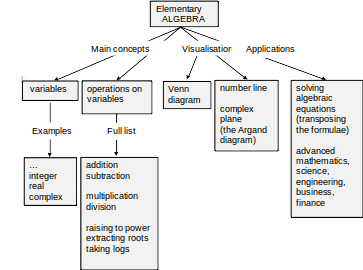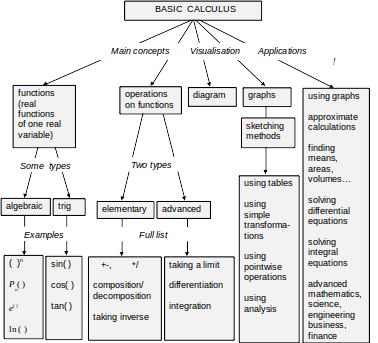Basic Calculus Unveiled

……………………………………………………

14 September 2013

I. INTRODUCTION

II. CONCEPT MAPS

III. LECTURES

Lecture 13. CALCULUS: Sequences, Limits and Series

13.1 Sequences

13.2 Limit of a sequence

13.3 Series

13.4 Instructions for self-study

Lecture 14. DIFFERENTIAL CALCULUS: Limits, Continuity and Differentiation of Real Functions of One Real Variable

14.1 Limits

14.2 Continuity of a function

14.3 Differentiation of a real function of one real variable

14.4 A historical note

14.5 Instructions for self-study

Lecture 15. DIFFERENTIAL CALCULUS: Differentiation (ctd.)

15.1 Differentiation Table

15.2 Differentiation Rules

15.3 Decision Tree for Differentiation

15.4 The higher order derivatives

15.5 The partial derivatives

15.6 Applications of differentiation

15.7 Instructions for self-study

Lecture 16. DIFFERENTIAL CALCUUS: Sketching Graphs Using Analysis

16.1 Stationary points

16.2 Increasing and decreasing functions

16.3 Maxima and minima

16.4 Convex and concave functions

16.5 Inflexion points

16.6 Sketching rational functions using analysis

16.7 Applications of rational functions and their graphs

16.8 Instructions for self-study

Lecture 17. Application of DIFFERENTIAL CALCULUS to Approximation of Functions: the Taylor and Maclaurin Series

17.1 Approximating a real function of a real variable using its first derivative

17.2 The Maclaurin polynomials

17.3 The Taylor polynomials

17.4 The Taylor series

17.5 The Maclaurin series

17.8 Instructions for self-study

Lecture 18. INTEGRAL CALCULUS: Integration of Real Functions of One Real Variable (Definite Integrals)

18.1 A definite integral

18.2 Notation

18.3 Comparison of a definite Integral and derivative

18.4 Examples of integrable functions

18.5 The Mean Value Theorem

18.6 A definite integral with a variable upper limit - function Φ(x)

18.7 The Fundamental Theorem of Calculus

18.8 Applications of integration

18.9 A historical note

18.10 Instructions for self-study

Lecture 19. INTEGRAL CALCULUS: Integration of Real Functions of One Real Variable (Indefinite Integrals)

19.1 The relationship between differentiation and integration

19.2 An indefinite integral

19.3 Finding an indefinite integral

19.4 Instructions for self-study

Lecture 20. INTEGRAL CALCULUS: Advanced Integration Methods

20.1 Integration of products of trigonometric functions

20.2 Integration by parts (integration of products of different types of functions)

20.3 Partial fractions

20.4 Integration of rational functions

20.5 Decision Tree for Integration

20.6 Instructions for self-study

Lecture 21. INTEGRAL CALCULUS: Applications of Integration

21.1 Mean value of a function

21.2 Electrical and mechanicsl systems

21.3 Rotational systems

21.4 Instructions for self-study

Lecture 22. Ordinary Differential Equations

22.1 Basic concepts

22.2 Order of a differential equation

22.3 Linearity or non-linearity of a differential equation

22.4 Differential equations with constant coefficients

22.5 Homogeneous and inhomogeneous ODEs

22.6 The first order linear homogeneous equation with constant coefficients

22.7 The initial value problems

22.8 Balance equations in chemical engineering

22.9 Ordinary differential equations with complex coefficients

IV. SUMMARIES

Algebra Summary

Functions Summary

Order of Operations Summary

Trigonometry Summary

Complex Numbers

Decision Tree For Solving Simple Equations

Sketching Graphs by Simple Transformations

Finding a Limit of a Sequence

Differentiation Summary

Integration Summary

V. GLOSSARY

VI. STUDY SKILLS FOR MATHS

VII. TEACHING METHODOLOGY (FAQs)

1. # INTRODUCTION

These notes are based on the lectures delivered by the author to engineering students of London South Bank University over the period of 16 years. This is a University of widening participation, with students coming from many different countries, many of them not native English speakers. Most students have limited mathematical background and limited time both to revise the basics and to study new material. A system has been developed to assure efficient learning even under these challenging restrictions. The emphasis is on systematic presentation and explanation of basic abstract concepts. The technical jargon is reduced to the bare minimum.

Nothing gives a teacher a greater satisfaction than seeing a spark of understanding in the students’ eyes and genuine pride and pleasure that follows such understanding. The author’s belief that most people are capable of succeeding in - and therefore enjoying - the kind of mathematics that is taught at Universities has been confirmed many times by these subjective signs of success as well as genuine improvement in students’ exam pass rates. Interestingly, no correlation had ever been found at the Department where the author worked between the students’ qualification on entry and graduation.

The book owns a lot to the authors’ students – too numerous to be named here - who talked to her at length about their difficulties and successes, e.g. see Appendix VII on Teaching Methodology. One former student has to be mentioned though – Richard Lunt – who put a lot of effort into making this book much more attractive than it would have been otherwise.

The author can be contacted through her website www.soundmathematics.com. All comments are welcome and teachers can obtain there the copy of notes with answers to questions suggested in the text as well as detailed Solutions to suggested Exercises. The teachers can then discuss those with students at the time of their convenience.

Good luck everyone!

1. # CONCEPT MAPS

Throughout when we first introduce a new concept (a technical term or phrase) or make a conceptual point we use the bold red font. We use the bold blue to verbalise or emphasise an important idea. Two major topics are covered in this course, Elementary Algebra and Basic Calculus.

Two major topics are covered in these Notes, Differential Calculus and Integral Calculus. You can understand these topics best if you first study the Notes on Elementary Algebra and Functions. Here is a concept map of Elementary Algebra.Here is a concept map of Basic Calculus. It is best to study it before studying any of the Calculus Lectures to understand where they are on the map. The better you see the big picture the faster you learn!